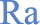## The Ra just-in-time compiler: a case study

Ra is functionally identical to R but provides just-in-time (JIT) compilation of loops and arithmetic expressions in loops. The result is faster code.

In this document we look at some variations of the same piece of R code to see some of the strengths and weaknesses of the JIT compiler. The code is the "Distribution of a determinant" on page 154 in Venables and Ripley S Programming. This example is also mentioned in An Introduction to R. It is introduced in Venables and Ripley as follows:

"Consider the problem of finding the distribution of the determinant of a 2 x 2 matrix where the entries are independent and uniformly distributed digits 0, 1, ..., 9. This amounts to finding all possible values of ac-bd where a, b, c and d are digits."

### The times, summarized

The table below summarizes timing results for the various solutions we will consider.
```Function         RTime   RaTime   Ratio     Notes
ms       ms    Ra/R

dd.for.c           240      230    0.96     original
dd.for.c1          240      err     err     c() prevents compile
dd.for.c2          240      201    0.84     nojit(val)

dd.for.prealloc    132       10    0.08     preallocate val
dd.for.tabulate    128        6    0.05     tabulate replaces table
dd.fast.tabulate  1.47     1.43    0.97     no loops
```
The tests were made on a 3GHz Pentium G on a Windows XP system using R 2.6.2 and Ra 1.0.6. The times are in milliseconds. Smaller time ratios mean that Ra is faster. The standard deviation of each number in the table is less than 5%. The code to reproduce these timing results is here.

### Variations on a theme

A first solution to the problem is
```    dd.for.c <- function()
{
val <- NULL
for (a in 0:9) for (b in 0:9) for (d in 0:9) for (e in 0:9)
val <- c(val, a*b - d*e)
table(val)
}
```
We enable just-in-time compilation by adding a jit(1) statement:
```    dd.for.c1 <- function()
{
jit(1) # enable just-in-time compilation
val <- NULL
for (a in 0:9) for (b in 0:9) for (d in 0:9) for (e in 0:9)
val <- c(val, a*b - d*e)
table(val)
}
```
but run into a problem. Under Ra, we get the error message:
```    Error: cannot change the length of a jitted variable
Tried to change "val" from length 1 to length 2
```
The problem is that the c() statement in the loop body is changing the length of val, and you are not allowed to change the size or type of a jitted variable. The environment has to be stable for the JIT compiler to optimize the code. A way around this is to specify nojit(val). Everything will get compiled as usual except expressions containing val:
```    dd.for.c2 <- function()
{
jit(1)
val <- NULL
nojit(val) # inhibit compilation of val
for (a in 0:9) for (b in 0:9) for (d in 0:9) for (e in 0:9)
val <- c(val, a*b - d*e)
table(val)
}
```
This function is about 20% faster under Ra. That's not much of an improvement. The for loops are indeed running faster but that improvement is swamped by the slowness of the call to c(). (In general, functions called from a JIT block --- such as c() --- are not compiled. Exceptions are mathematical functions such as sin.) So we get rid of the c() by preallocating val:
```    dd.for.prealloc <- function()
{
jit(1)
val <- double(10000)    # preallocate val
nval <- 0
for (a in 0:9) for (b in 0:9) for (d in 0:9) for (e in 0:9)
val[nval <- nval + 1] <- a*b - d*e
table(val)
}
```
The function is now faster under both R and Ra, but with Ra about 13 times faster than R because of JIT compilation.

### Replacing table with tabulate

We can replace the call to table() with the faster function tabulate():
```    dd.for.tabulate <- function()
{
jit(1)
val <- double(10000)
nval <- 0
for (a in 0:9) for (b in 0:9) for (d in 0:9) for (e in 0:9)
val[nval <- nval + 1] <- a*b - d*e
tabulate(val)   # was table()
}
```
With this, Ra runs about 21 times faster than R.

### Avoiding loops

Venables and Ripley give a solution that avoids loops altogether:
```    dd.fast.tabulate.jit <- function()
{
jit(1)
val <- outer(0:9, 0:9, "*")
val <- outer(val, val, "-")
tabulate(val)
}
```
This solution is the fastest of all. The Ra time is about the same as the R time because there are no loops to optimize. The jit(1) statement has no effect.

### Some conclusions

We should not draw too many conclusions from one case study, but it seems safe to say the following.

"Vectorization" (i.e. getting rid of loops) usually gives you the fastest code.

The JIT compiler can give substantial performance improvements for looped arithmetic. It is useful when using loops is a natural or convenient expression of the problem, or when code cannot be vectorized.